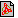Cím: Practice problems for the entrance exam Szerző(k): Imre Rábai Füzet: 2003/októberi melléklet, 24. oldalPDF file Témakör(ök): Felvételi előkészítő feladatsor

A szöveg csak Firefox böngészőben jelenik meg helyesen. Használja a fenti PDF file-ra mutató link-et a letöltésre.

In memory of György Geréb, psychologist and college professor

1. A trapezium has both an inscribed circle and a circumscribed circle. One of the parallel sides is 10 units long and the radius of the incircle is $\varrho =5\sqrt[]{3}$ units. What percentage of the area of the trapezium is the area of the incircle?

2. Two cities are $560\phantom{\rule{0.5ex}{0ex}}\text{km}$ apart. A car takes 1 hour less to cover this distance than another car because its speed is $10\phantom{\rule{0.5ex}{0ex}}\frac{\text{km}}{\text{h}}$ greater than that of the other one. Find the speeds of the two cars.

3. The points $P\left(b,-2\right)$ and $Q\left(16b,6\right)$ lie on the graph of the logarithm function $f:x↦\text{log}{}_{a}x$, $x\in {\text{R}}^{+}$ ($a>0$, $a\ne 1$). Determine the values of $a$ and $b$ and the equation of the line $PQ$.

4. Find the domain and range of the expression
 $\begin{array}{c}\frac{\text{sin}2x+2\text{sin}x}{\text{sin}2x-2\text{sin}x}\cdot \text{tan}{}^{2}\frac{x}{2}.\end{array}$

5. Find the equation of the circle of radius $\sqrt[]{8}$ passing through the point $P\left(0,2\right)$ and touched from the outside by the circle of equation
 $\begin{array}{c}{x}^{2}+{y}^{2}+2x+6y+8=0.\end{array}$

6. Solve the following inequality on the set of real numbers:
 $\begin{array}{c}2\sqrt[]{\text{log}{}_{3}x}+\sqrt[]{\text{log}{}_{x}3}\ge 3.\end{array}$

7. A convex hexagon with three sides of length $2a$ and three sides of length $5a$ is inscribed in a circle of radius $\sqrt[]{13}$. Find the area of the hexagon.

8. The first term of a sequence is ${a}_{1}=1$, and
 $\begin{array}{c}{a}_{n+1}=\left(1-\frac{1}{{\left(n+2\right)}^{2}}\right)\cdot {a}_{n}\end{array}$
for $n\ge 1$. Express (in closed form) the $n$th term of the sequence and the product of the first $n$ terms.